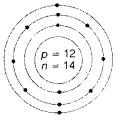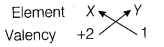# What is the valency of the element and why?

1. An element X has an atomic number = 12 and mass number = 26. Draw a diagram showing the distribution of electrons in the orbits and the nuclear composition of the neutral atom of the element. What is the valency of the element and why?
2. If this element X combines with another element Y whose electronic configuration is 2,8,7. What will be the formula of the compound thus formed? State how did you arrive at this formula.
1. Atomic number =12
Mass number =26
Atomic structure of X
Electronic configuration = 2, 8, 2Nuclear composition
Number of protons = 12
Number of neutrons =26-12 = 14 (1)
Valency = 2 (1/2)
Because it can donate 2 electrons easily to complete its octet and become stable.
2. Valency of the element Y would be 1, as it can gain 1 electron to become stable. When it combines with the element X of valency 2, the compound formed will be XY2.Formula of the compound would be XY2.